## Solving system with constraints ...SYSTEMATIC APPROACH TO LIFTING EYE DESIGN

MosesNomenclature Ab  = Required bearing area, sq in. (mm2) As  = Required shear area at hole, sq in. (mm2) Aw = Required cheek plate weld area, sq in. (mm2) b     = Distance from center of eye to the cross section, in. (mm) C    = Percentage distance of element from neutral axis D    = Diameter of lifting pin, in. (mm) e     = Distance between edge of cheek plate and edge of main plate, in. (mm) Fa   = Allowable normal stress, ksi (kN/mm2) Fv   = Allowable shear stress, ksi (kN/mm2) Fw  = Allowable shear stress for weld electrodes, ksi (kN/mm2) Fy   = Yield stress, ksi (kN/mm2) fa   =  Computed axial stress, ksi (kN/mm2) fb   =  Computed bending stress, ksi (kN/mm2) fmax = Maximum principal stress, ksi(kN/mm2) fv    = Computed shear stress, ksi (kN/mm2) g     = Distance between edge of cheek plate and main structure, in. (mm) h    = Length of lifting eye at any cross section between A-A and C-C, in. (mm) n   = Total number of lifting eyes used during the lift P  = Design load per lifting eye, kips (kN) R  = Radius to edge of lifting eye, in. (mm) Rh  = Radius of hole, in. (mm) r    = Radius of cheek plate, in. (mm) S   = Safety factor with respect to allowable stresses s  = Cheek plate weld size, in. (mm) T  = Total plate thickness, in. (mm) Tp  = Main plate thickness, in (mm) t    = Thickness of each cheek plate, in (mm) te  = Cheek plate weld throat, in. (mm) W  = Total lift weight of structure, kips (kN) α  =  Angle of taper, deg. β  =  Angle between vertical and lifting sling, deg. θ  =  Angle between attaching weldment and lifting sling, deg.The design load for each lifting eye is given by:

 >>In the above equation, n refers to number of lifting eyes to used for the lift, S is the safety factor with respect to allowable stresses, W is the total weight to be lifted, and β is the angle between the vertical direction and the lifting sling.This analysis applies only to lifting eyes shaped like the one in Fig. 1. For other shapes, the designer should re-evaluate the equations.

Radius of liftimg eye hole will depend upon the diameter of the pin, D, used in the lifting shackle. It is recommeded that the hole diameter not greater than 1 / 16 in. (2 mm) larger than tha pin diameter. The required bearing area for the pin is

 >where Fy is the yield stress. This equation is based on allowable stresses as definde in Ref. 1, which considers stress concentrations in the vicinity of the hole. The designer may choose to use a technique which determines the stresses at the hole and should appropriately adjust the allowable stresses. The total plate thickness is then given by

 >At this point, if the thickness, T, is too large to be economically feasible, it may be desirable to use cheek plates (Fig.2) around the hole in order to sustain the bearing stresses. In this case, the above thickness, T, is divided into a main plate of thickness Tp and two cheek plates each of thickness t:

 >It is recommended that t be less than Tp to avoid excessive welding. The radius to the edge of lifting eye plate and the radii of the cheek plates, if they are used, are governed by the condition that the pin cannot shear through these plates. The required area for shear is

 >It is possible to compute the required radii by equating the shaering area of the cheek plates plus the shearing area of the main plate to the total shear area. Theis a degree of uncertianty in choosing the appropriate shearing area. Minimum areas are used in the following equation, therefore, leading to conservative values for the radius of the main plate, R, and the radius of the cheek plate, r,

 >>where Rh is the radius of the hole and e is the distance between the edge of the cheek plate and the edge of the main plate (Fig. 2). This difference should be large enough to allow space for welding the cheet plate to the main plate. A reasonable value for e is 1.5*t. It should be noted that the above equations assume there are two cheek plates. If cheek plates are not used, then simply let t equal zero and use Eq. 6 to determine R.

It is not necessary to check tension on this net section, since the allowable stress for shear is 0.4*Fy (Eq. 5); whereas the allowable stress for tension on a net section at a pin hole is given as 0.45*Fy (Ref. 1) which is greater than for shear. Size of weld between the cheek plates and the main plate can be determined as follows. The necessary weld area per cheek plate is

 >where Fw is the allowable shear stress for the welding electrodes. The weld thickness, te is given by

 >For a manual weld the size, s is given by

 >To assure that this weld size is large enough to insure fusion and minimize distortion, it should be greater than the AISC suggested Minimum Fillet Weld Sizes (Ref. 1).

The axial stress due to uniform tension along a section is

 >where h is the length of the section. The elemental bending stress which is distributed linearly along the section may be expressed as

 >where C represents the distance of an element from the neutral axis and b is the distance from the center of the eye to the cross section. The shearing stress varies parabolically for section between A-A and B-B and is given as

 >It is felt that Eq. 13 (i.e., parabolic shear stress distribution) is applicable to the cross sections between A-A and B-B and does not apply to the cross sections between B-B and C-C in the area of the taper. The taper creates discontinuities on the shear plane, which result in significantly large shear stress concentratons along the edge of the taper coincident to point of maximum bending stress. This problem will be addressed ina subsequent section of this article.

The maximum principal stress that exists on an element is given by

 >or after dividing by the maximum allowable normal (i.e., tension) stress, Fa, gives a ratio that must be less than unity, where Fa has been taken as 0.6*Fy. A similar analysis for the maximum shear stress on the element yields

 >>>>>The designer should now select several critical elements throughout the plate and apply the restrains of Eq. 16 and 17 to obtain a required minimum length for the selected cross section. Eq 18 through 21 apply for an element at the neutral axis of the section. C will be zero and Eq. 11, 13 and 16 reduce to

 >>An element at the end of the section will be subjected to bending stresses but not shearing stresses.

For this case C = 0.5 and Eq. 16  becomes

 >Using the quadratic formular to solve for h yields

 >The largest value of h predicted by Eqs. 19, 21 and 23 can be used as a first estimate for the length of the cross section; however, intermediate elements, that is, between the edge and the center of the cross section, should also be checked to determine the appropriate length, h, of the section under consideration.

Cross sections A-A and B-B should be analyzed using the above approach. The designer should use his own discretion to select other cross sections for analysis.At cross section A-A, the lifting eye is assumed to be welded with complete penetration to the support structure. Once length, h, is determined, the angle of taper, α, should be investigated. It can be shown that normal stress and shear stress are related by

 >Minimum required length, h, for cross sections between B-B and C-C can be computed by calculating the maximum shear stress for the most critical element of the cross section, which occurs at the tapered surface. It can be shown that the maximum principal stress would not control the required length, h. Using Eqs. 11 and 12 in conjunction with Eq 24, the maximum shear stress yields the following:

 >If the above inequality is not satisfied, the angle of the taper, α, must be adjusted.

The adequacy of the structure to which the lifting eye is to be attached should be checked to verify that it is capable of sustaining the loads from the lifting eye.

In some instances, it may be justifiable to use a more sophisticated technique for analyzing the lifting eye as well as the supporting structure.Input Variables

 >>>>>>>>>>>>>>OutputGood Evening Everybody,

Could any one help me with the attached file. I'm trying to solve 9 equations with 9 unknowns with many constraints, I'm getting no output from Maple. Please help.

Regards,

Moses

## How do I solve this system of ODEs in Maple?...

I'm trying to solve a system of 4 ODE's.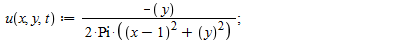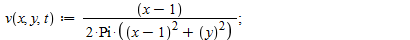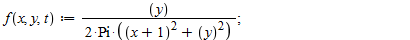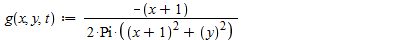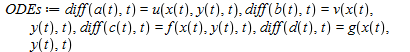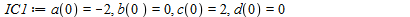however I have 4 equations and six unknowns. I dont know how else to describe the functions a,b,c,d

cause these just represent vector valued functions at points (x1,y1) and (x2,y2) where i have chosing (x1,y1)=(-1,0) and (x2,y2) = (1,0)

I have that

dx1/dt = (u,v)

dx2/dt=(f,g)

I know that if i graph these functions I should get vertical lines, but I keep getting circles if I instead consider a(t) to be x(t) and b(t) to be y(t)...

I need to solve this system and plot it but i am misinterpreting something somewhere..

## Conditional statements...

I have the following question:

Illustrate how the sequence N->R de fined by n ->n^2/n^2 + 31n + 228 can be shown to be
within a given epsilon > 0 of its limiting value x0.
(a) use an appropriate conditional statement to find N such that abs (xn -􀀀 x0) < epsilon for every n>=N
and produce an appropriate list of the data points (n,xn) to illustrate
this

I found N but without using any CONDITIONAL STATEMENT.Can you help me find N using IF FOR WHILE?

## Matrix Procedure Query...

Hi. I'm hacing trouble writing a maple procedure for the question below, can anyone help?

Write a maple procedure which takes as its input the vectoeat u1 and u2 and the eigenvectors lambda1 and lambda2 where u1,u2 are element of R^2 and the lambdas are real numbers.

If u1,U2 is linearly independent then the output is the matrix A an element of R^2x2 with the property that Au1= lambda1u1 and AU2=lambda2u2;

if u1,u2 is linearly dependent then the output is the statement "not an eigenbasis".

I I then have two inputs which I have to do but I'm not sure on how to write the procedure. Any help will be much appreciated.

Thanks :)

## Why different results?...

Dear all,

I developed a program to solve f(x, y) = 0 and g(x, y) = 0, I obtained as results (x=2.726, y=2.126) . running the same program another time it gives (x=2.762, y=1.992). how to explain this?

> fsolve({f(x, y) = 0, g(x, y) = 0}, {x = 0 .. infinity, y= 0 .. infinity});

## How to use Greek alphabet in Maple?...

hello

I have recently install Maple17 on my computer (Windows10) and I need to use some Greece alphabet such as ß but I look everywhere in maple's icon and I just could find capital Greece alphabet.

does anybody know how can I find those?

## Execute a maple file from another maple file and d...

I have several *.mw files which I have built seperately. Now, for readability reasons, I would like to create a Master document, which will ues the first files, and then generate a PDF document.
I tried the read command, but it does not recognize the files.

Any help is appreciated.

## How to simplify it?...

can someone tell me how can I derive and solve d(g1(x))=0 a complicated function as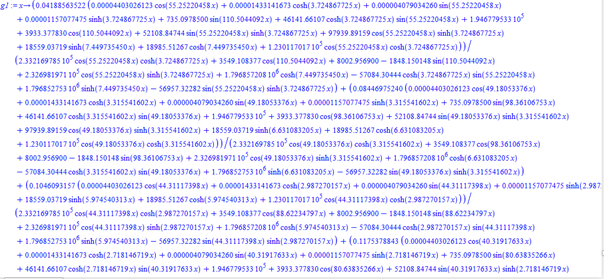I tried with the instructions simplify and combine but without results.

## Ploottools in Maple 17...

Hello all!

I did

with(plottools):

display(pieslice([0,0],5,Pi/4..7Pi/4,color=yellow))

but I don't know how to do "Animating Pacman". Can you help me? I read it on Mapleprimes but I don't really understand. Can you explain it to me? Thank you so much! Sorry, My English is not very good## Error, (in dsolve/numeric/bvp/convertsys) unable t...

Hello i want to solve the differential equation but i have these problem i don't understand  why !?

restart;
with(LinearAlgebra):
with(student):
P:=180000:
Vt:=P/m;
m:=1.4787880*10^5-t*606.06:
g:=9.81:
T:=176:
pi:=evalf(Pi):

Euler_x := -(diff(lambda3(t), t)) = 0;
Euler_y := -(diff(lambda4(t), t)) = 0;
Euler_Vx := -lambda3(t)-(diff(lambda1(t), t)) = 0;
Euler_Vy := -lambda4(t)-(diff(lambda2(t), t)) = 0;
Euler_theta := lambda1(t)*sin(theta)-lambda2(t)*cos(theta) = 0;
transversalnost:=y(t)-lambda1(t)*Vt*cos(theta)-lambda2(t)*Vt*sin(theta)+lambda2(t)*g-lambda3(t)*Vx(t)-lambda4(t)*Vy(t)=0;

transversalnost:=eval(transversalnost,t=176);

sys:={Euler_x, Euler_Vx, Euler_y, Euler_Vy, theta=arctan(lambda2(t)/lambda1(t)), diff(Vx(t),t)=Vt*cos(theta), diff(Vy(t),t)=Vt*sin(theta)-g, diff(y(t),t)=Vy(t), diff(x(t),t)=Vx(t),x(0)=0, y(0)=0.302, lambda1(0)=0, lambda2(0)=0, lambda3(0)=0, lambda4(0)=0,transversalnost, x(176)=120};

ans:=dsolve(sys, numeric, output=listprocedure):

Error, (in dsolve/numeric/bvp/convertsys) unable to convert to an explicit first-order system

## How to paste a MAPLE text ...

into the "Ask a Question" window?

Actually I want to ask something else.

## How to iterate the wavlet transform?...

Take for instance the signal [1,0,-3,2,1,0,1,2] (considered in Wavelets for Kids, Vidakivic & Mueler, AMS, 1991).

I want to anlalyse it down to the level zero with Haar wavelets. It seems that MAPLE's commnad only applies once.

## Is there any other way?...

Hello maple experts,

please is there any other way of solving this problem Wht.mw

Some complained that the graphs do not satisfy the boundary conditions smoothly.

Thanks.

## evaluating forever......

hello people

I have this computation which has to do with my undergraduate project and each time I compute some work (vary parameters), it seems to evaluate forever. although my computer isn't recent and has 2GB of RAM the computation didn't seem to me as much of a task for it. computation works fine with some parameters as 0 but the moment I change it to a natural number, it evaluates forever.

Is there anyway I could speed up computation in maple? or do I just need a faster computer? but I have a dead line for next week. can I upload my worksheet for someone here to help me execute?